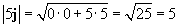How to Multiply Vectors - Scalar (dot) product

How to Multiply Vectors

Key Terms

• Unit vector
• Scalar (dot) product
•

Objectives

• Define the concept of unit vectors
• Use unit vectors to represent vectors algebraically
• Define multiplication of a scalar and a vector
• Use the scalar product to calculate the length of a vector

• In this article, we will look at another representation of vectors, as well as the basics of vector multiplication.

Unit Vectors

Although the coordinate form for representing vectors is clear, we can also represent them as algebraic expressions using unit vectors. In our standard rectangular (or Euclidean) coordinates (x, y, and z), a unit vector is a vector of length 1 that is parallel to one of the axes. In the two-dimensional coordinate plane, the unit vectors are often called i and j, as shown in the graph below. For three dimensions, we add the unit vetor k corresponding to the direction of the z-axis. These vectors are defined algebraically as follows.

i = (1, 0) or (1, 0, 0)

j = (0, 1) or (0, 1, 0)

k = (0, 0, 1)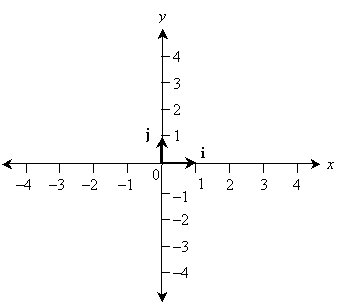Before we present an algebraic representation of vectors using unit vectors, we must first introduce vector multiplication--in this case, by scalars.

Vector Multiplication by Scalars

Multiplication involving vectors is more complicated than that for just scalars, so we must treat the subject carefully. Let's start with the simplest case: multiplying a vector by a scalar. Below is the definition for multiplying a scalar c by a vector a, where a = (x, y). (Again, we can easily extend these principles to three dimensions.)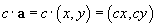Scalar multiplication is commutative, so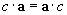. But what does this multiplication mean? As it turns out, multiplication by a scalar c has the effect of extending the vector's length by the factor c. This is most clearly seen with unit vectors, but it applies to any vector. (Multiplication by a negative scalar reverses the direction of the vector, however.) The graph below shows some examples using c = 2. (Recall that the location of a vector doesn't affect its value.)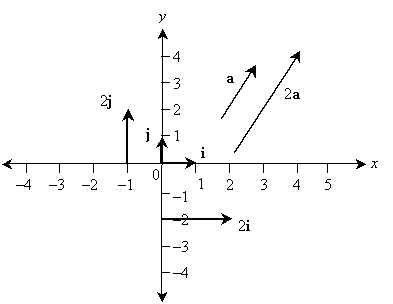Practice Problem: Given a vector a = (3, 1), find a vector in the same direction as a but twice its length.

Solution: When we multiply a vector by a scalar, the direction of the product vector is the same as that of the factor. The only difference is the length is multiplied by the scalar. So, to get a vector that is twice the length of a but in the same direction as a, simply multiply by 2.

2a = 2 • (3, 1) = (2 • 3, 2 • 1) = (6, 2)

Algebraic Representation of Vectors

We can use scalar multiplication with vectors to represent vectors algebraically. Note that any two-dimensional vector v can be represented as the sum of a length times the unit vector i and another length times the unit vector j. For instance, consider the vector (2, 4). Apply the rules of vectors that we have learned so far:

(2, 4) = (2, 0) + (0, 4) (addition rule for vectors)

(2, 4) = 2 • (1, 0) + 4 • (0, 1) (multiplication rule for scalars and vectors)

(2, 4) = 2i + 4j

Graphically, we are adding two vectors in the unit directions to get our arbitrary vector.

Interested in learning more? Why not take an online Precalculus course?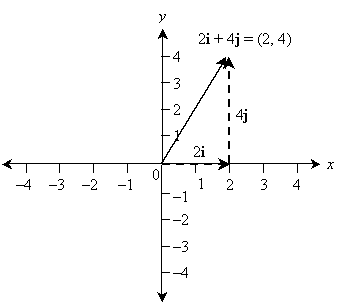Note that the unit vectors act almost identically to variables. Thus, we can add two vectors a and b as follows.

a = 3i – 2j b = i + 3j

a + b = (3i – 2j) + (i + 3j) = 3i + i – 2j + 3j = 4i + j

This representation provides more flexibility than the coordinate representation, but it is equivalent.

Practice Problem: Calculate the sum and difference (t - u) of the vectors t = -2i + 3j and u = 6i - 4j.

Solution: We can solve this problem algebraically quite easily.

t + u = (-2i + 3j) + (6i - 4j) = 4i - j = (4, -1)

t - u = (-2i + 3j) - (6i - 4j) = -2i + 3j - 6i + 4j = -8i + 7j = (-8, 7)

Vector Multiplication: The Scalar (Dot) Product

Multiplication of two vectors is a little more complicated than scalar multiplication. Two types of multiplication involving two vectors are defined: the so-called scalar product (or "dot product") and the so-called vector product (or "cross product"). For simplicity, we will only address the scalar product, but at this point, you should have a sufficient mathematical foundation to understand the vector product as well. The scalar product (or dot product) of two vectors is defined as follows in two dimensions. As always, this definition can be easily extended to three dimensions-simply follow the pattern. Note that the operation should always be indicated with a dot (•) to differentiate from the vector product, which uses a times symbol (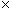)--hence the names dot product and cross product.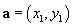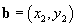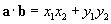The meaning of this product may not be entirely clear to you at this point, however. We can illustrate by looking at a simple case: the scalar product of an arbitrary vector v and the unit vectors i and j.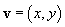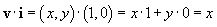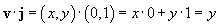Thus, vi is the "part" of vector v in the direction of I.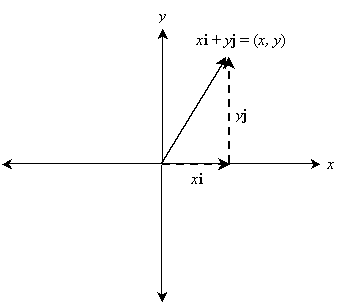This explanation only works, however, for vectors of length 1. When two arbitrary vectors are multiplied, the scalar product has a similar meaning, but the magnitude of the number is a little different. We won't go into this in any further depth, but we can consider a special case where the scalar product yields valuable information.

The Length of a Vector

Consider the case of a scalar product of a vector v with itself.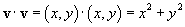Let's look at this situation graphically.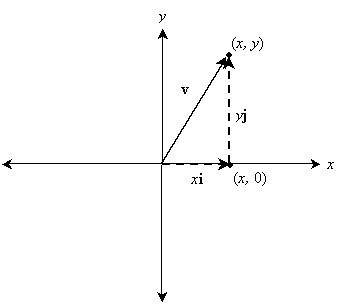The result here is a right triangle with a horizontal leg of length x and a vertical leg of length y. These lengths correspond to the lengths of the component vectors xi and yj, respectively. But we know from the Pythagorean theorem that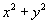is the square of the length of vector v. Not coincidentally, this is the same as the scalar product of v with itself. Thus, the length of any vector v, written as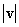(or sometimes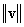) is the square root of the scalar product.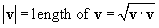In the simple case of the unit vectors,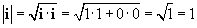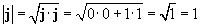These simple cases help verify this interpretation of the scalar product.

Practice Problem: Calculate the lengths of the following vectors.

a.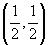b. 3i + 2jk c. (2, –1) d. 5j

Solution: In each case, simply take the square root of the scalar product of the vector with itself. The result is the length of the vector in each case. For part b, simply extend the definition of the scalar product to three dimensions.

a.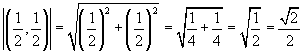b.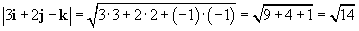c.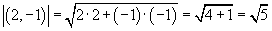d.